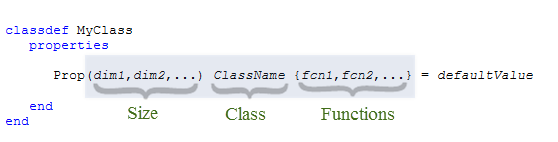Main Content

## 验证属性值

### 类定义中的属性验证

MATLAB® 属性验证使您能够对属性值进行特定限制。您可以使用验证来约束属性值的类和大小。此外，您可以使用函数来建立属性值必须符合的条件。MATLAB 定义了一组验证函数，您也可以编写自己的验证函数。

#### 验证语法• 大小 - 每个维度的长度，指定为正整数或冒号。冒号表示该维度中允许任何长度。赋给属性的值必须符合指定的大小或与指定的大小兼容。有关详细信息，请参阅Property Size Validation

• 类 - 单个 MATLAB 类的名称。赋给属性的值必须属于指定的类或可转换为指定的类。使用 MATLAB 类或 MATLAB 支持的任何外部定义的类，但 Java® 和 COM 类除外。有关详细信息，请参阅Property Class Validation

• 函数 - 以逗号分隔的验证函数名称列表。MATLAB 在应用任何可能的类和大小转换后，将赋给属性的值传递给每个验证函数。如果验证失败，验证函数会引发错误，但不会返回值。有关详细信息，请参阅属性验证函数

有关 MATLAB 验证函数的列表，请参阅属性验证函数

#### 使用属性验证

MATLAB 类型转换规则适用于属性验证。例如，MATLAB 可以对数值类型进行强制转换。因此，将属性值限制为特定的数值类型（如双精度）不会阻止使用其他数值类型对属性赋值。

### 使用属性验证的示例类

`ValidateProps` 类定义三个属性并进行验证。

```classdef ValidateProps properties Location(1,3) double {mustBeReal, mustBeFinite} Label(1,:) char {mustBeMember(Label,{'High','Medium','Low'})} = 'Low' State(1,1) matlab.lang.OnOffSwitchState end end```
• `Location` 必须为 `double` 类的 1×3 数组，其值是有限实数。

• `Label` 必须为 `char` 向量，包含 `'High'``'Medium'``'Low'` 之一。

• `State` 必须为 `matlab.lang.OnOffSwitchState` 类的枚举成员（`off``on`）。

#### 实例化时的验证

```a = ValidateProps ```
```a = ValidateProps with properties: Location: [0 0 0] Label: 'Low' State: off```

• `Location` 属性值初始化为 `[0 0 0]`，以满足大小和类要求。

• `Label` 属性设置为默认值 `'Low'`。默认值必须为允许的值集的成员。空的 `char` 隐式默认值会导致错误。

• `State` 属性设置为 `matlab.lang.OnOffSwitchState` 类定义的 `off` 枚举成员。

### 验证的顺序

• 类验证 - 此验证可导致转换为不同类，例如将 `char` 转换为 `string`。属性赋值遵循 MATLAB 对数组的强制转换规则。

• 大小验证 - 此验证可导致大小转换，例如标量扩展或列向量到行向量的转换。对指定大小验证的属性进行赋值时，其行为与 MATLAB 数组的赋值行为相同。有关索引赋值的信息，请参阅数组索引

• 验证函数 - MATLAB 按照从左到右的顺序将类验证和大小验证的结果传递给每个验证函数。在调用完所有验证函数之前可能会发生错误，从而结束验证过程。

• set 方法 - MATLAB 在调用属性 set 方法（如果为属性定义了该方法）之前执行属性验证。通过属性 set 或 get 方法为属性赋值时不会再次应用验证。通常，您可以使用属性验证来替换属性 set 方法。

#### 属性验证错误

`ValueProp` 类使用大小、类和函数验证来确保对 `Value` 属性的赋值是非负的双精度标量。

```classdef ValueProp properties Value(1,1) double {mustBeNonnegative} = 0 end end```

`a.Value = {10,20};`
```Error setting property 'Value' of class 'ValueProp': Invalid data type. Value must be double or be convertible to double.```

`a.Value = [10 20];`
```Error setting property 'Value' of class 'ValueProp': Size of value must be scalar.```

`a.Value = -10;`
```Error setting property 'Value' of class 'ValueProp': Value must be nonnegative.```

### 加载操作期间的验证

```classdef MonthTemp properties AveTemp cell end end```

```a = MonthTemp; a.AveTemp = {'May',70};```

`save TemperatureFile a`

```classdef MonthTemp properties AveTemp containers.Map end end```

```load TemperatureFile a a.AveTemp ans = Map with properties: Count: 0 KeyType: char ValueType: any ```

Download ebook